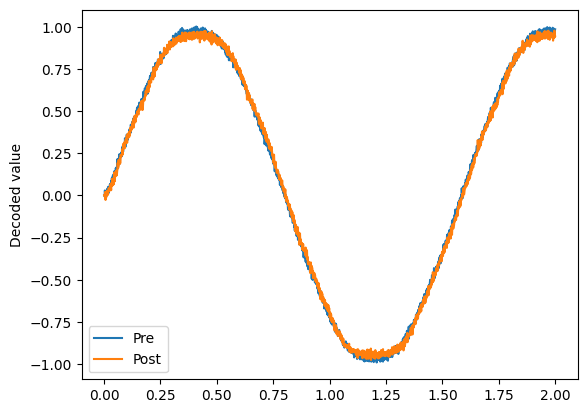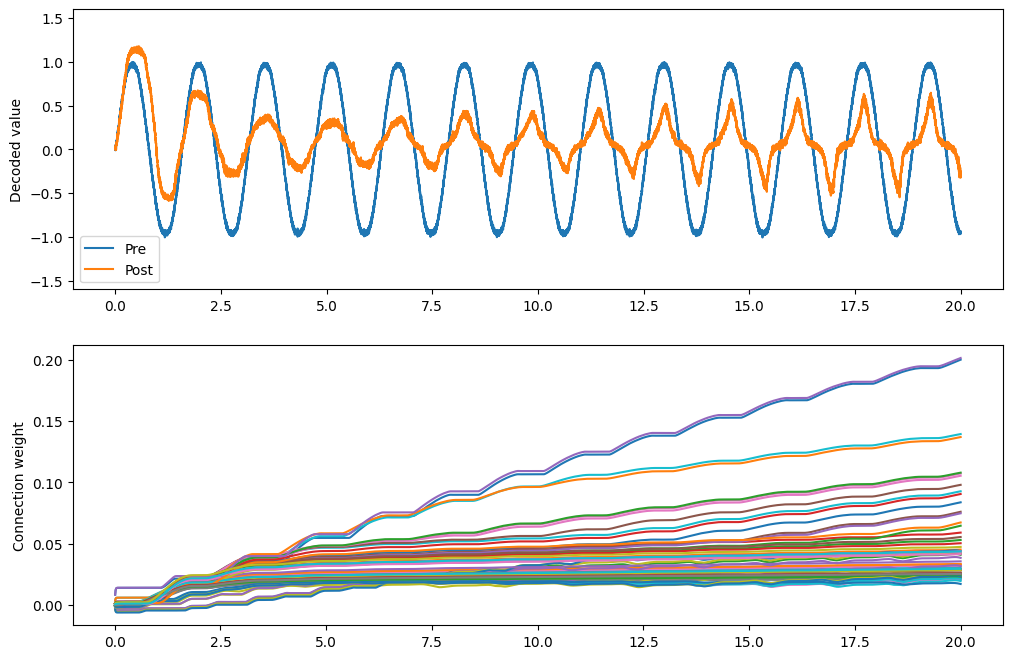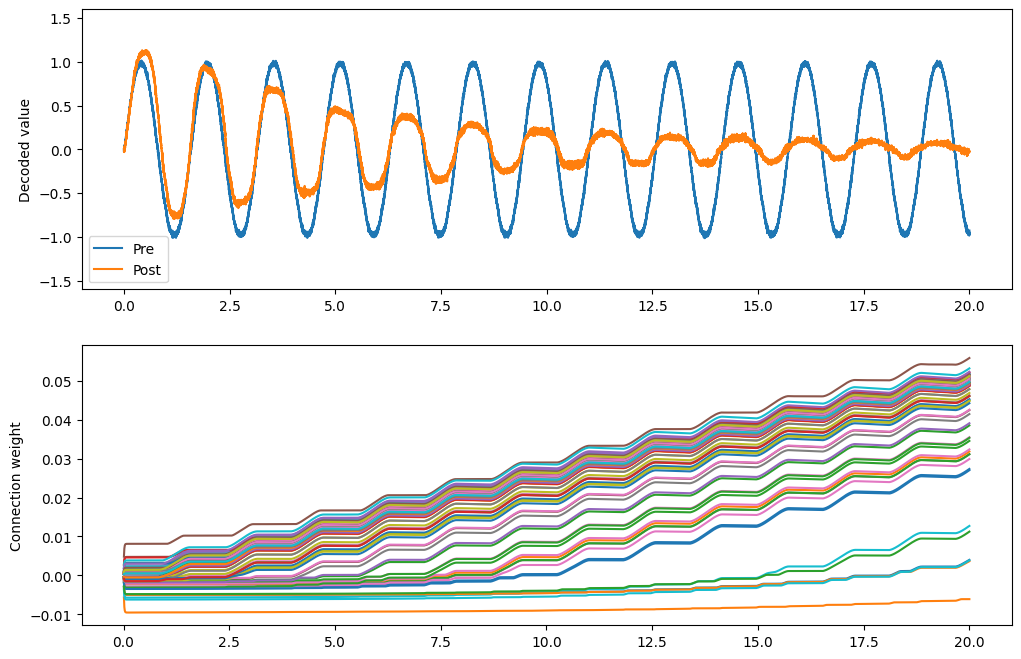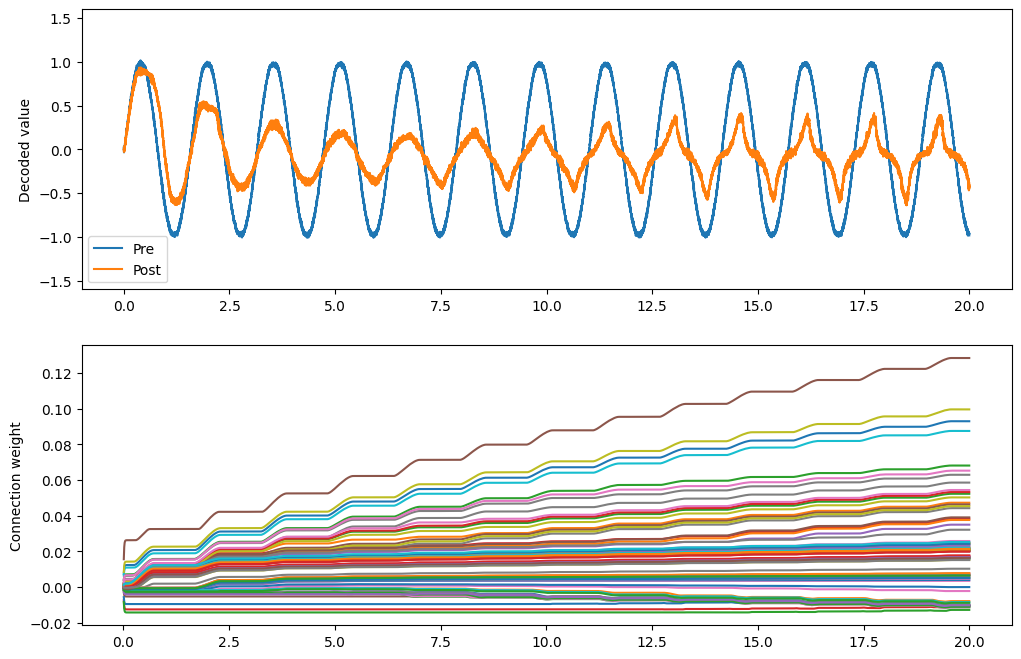Note

This documentation is for a development version. Click here for the latest stable release (v3.2.0).

# Unsupervised learning¶

When we do error-modulated learning with the nengo.PES rule, we have a pretty clear idea of what we want to happen. Years of neuroscientific experiments have yielded learning rules explaining how synaptic strengths change given certain stimulation protocols. But what do these learning rules actually do to the information transmitted across an ensemble-to-ensemble connection?

We can investigate this in Nengo. Currently, we’ve implemented the nengo.BCM and nengo.Oja rules.

:

import numpy as np
import matplotlib.pyplot as plt

%matplotlib inline

import nengo

:

print(nengo.BCM.__doc__)


Bienenstock-Cooper-Munroe learning rule.

Modifies connection weights as a function of the presynaptic activity
and the difference between the postsynaptic activity and the average
postsynaptic activity.

Notes
-----
The BCM rule is dependent on pre and post neural activities,
not decoded values, and so is not affected by changes in the
size of pre and post ensembles. However, if you are decoding from
the post ensemble, the BCM rule will have an increased effect on
larger post ensembles because more connection weights are changing.
In these cases, it may be advantageous to scale the learning rate
on the BCM rule by 1 / post.n_neurons.

Parameters
----------
learning_rate : float, optional
A scalar indicating the rate at which weights will be adjusted.
pre_synapse : .Synapse, optional
Synapse model used to filter the pre-synaptic activities.
post_synapse : .Synapse, optional
Synapse model used to filter the post-synaptic activities.
If None, post_synapse will be the same as pre_synapse.
theta_synapse : .Synapse, optional
Synapse model used to filter the theta signal.

Attributes
----------
learning_rate : float
A scalar indicating the rate at which weights will be adjusted.
post_synapse : .Synapse
Synapse model used to filter the post-synaptic activities.
pre_synapse : .Synapse
Synapse model used to filter the pre-synaptic activities.
theta_synapse : .Synapse
Synapse model used to filter the theta signal.


:

print(nengo.Oja.__doc__)


Oja learning rule.

Modifies connection weights according to the Hebbian Oja rule, which
augments typically Hebbian coactivity with a "forgetting" term that is
proportional to the weight of the connection and the square of the
postsynaptic activity.

Notes
-----
The Oja rule is dependent on pre and post neural activities,
not decoded values, and so is not affected by changes in the
size of pre and post ensembles. However, if you are decoding from
the post ensemble, the Oja rule will have an increased effect on
larger post ensembles because more connection weights are changing.
In these cases, it may be advantageous to scale the learning rate
on the Oja rule by 1 / post.n_neurons.

Parameters
----------
learning_rate : float, optional
A scalar indicating the rate at which weights will be adjusted.
pre_synapse : .Synapse, optional
Synapse model used to filter the pre-synaptic activities.
post_synapse : .Synapse, optional
Synapse model used to filter the post-synaptic activities.
If None, post_synapse will be the same as pre_synapse.
beta : float, optional
A scalar weight on the forgetting term.

Attributes
----------
beta : float
A scalar weight on the forgetting term.
learning_rate : float
A scalar indicating the rate at which weights will be adjusted.
post_synapse : .Synapse
Synapse model used to filter the post-synaptic activities.
pre_synapse : .Synapse
Synapse model used to filter the pre-synaptic activities.



## Step 1: Create a simple communication channel¶

The only difference between this network and most models you’ve seen so far is that we’re going to set the decoder solver in the communication channel to generate a full connection weight matrix which we can then learn using typical delta learning rules.

:

model = nengo.Network()
with model:
sin = nengo.Node(lambda t: np.sin(t * 4))

pre = nengo.Ensemble(100, dimensions=1)
post = nengo.Ensemble(100, dimensions=1)

nengo.Connection(sin, pre)
conn = nengo.Connection(pre, post, solver=nengo.solvers.LstsqL2(weights=True))

pre_p = nengo.Probe(pre, synapse=0.01)
post_p = nengo.Probe(post, synapse=0.01)

:

# Verify that it does a communication channel
with nengo.Simulator(model) as sim:
sim.run(2.0)

plt.figure()
plt.plot(sim.trange(), sim.data[pre_p], label="Pre")
plt.plot(sim.trange(), sim.data[post_p], label="Post")
plt.ylabel("Decoded value")
plt.legend(loc="best")

:

<matplotlib.legend.Legend at 0x7fe45be4dd90>## What does BCM do?¶

:

conn.learning_rule_type = nengo.BCM(learning_rate=5e-10)
with model:
weights_p = nengo.Probe(conn, "weights", synapse=0.01, sample_every=0.01)

:

with nengo.Simulator(model) as sim:
sim.run(20.0)

:

plt.figure(figsize=(12, 8))
plt.subplot(2, 1, 1)
plt.plot(sim.trange(), sim.data[pre_p], label="Pre")
plt.plot(sim.trange(), sim.data[post_p], label="Post")
plt.ylabel("Decoded value")
plt.ylim(-1.6, 1.6)
plt.legend(loc="lower left")
plt.subplot(2, 1, 2)
# Find weight row with max variance
neuron = np.argmax(np.mean(np.var(sim.data[weights_p], axis=0), axis=1))
plt.plot(sim.trange(sample_every=0.01), sim.data[weights_p][..., neuron])
plt.ylabel("Connection weight")

:

Text(0, 0.5, 'Connection weight')The BCM rule appears to cause the ensemble to either be on or off. This seems consistent with the idea that it potentiates active synapses, and depresses non-active synapses.

As well, we can show that BCM sparsifies the weights. The sparsity measure below uses the Gini measure of sparsity, for reasons explained in this paper.

:

def sparsity_measure(vector):  # Gini index
# Max sparsity = 1 (single 1 in the vector)
v = np.sort(np.abs(vector))
n = v.shape
k = np.arange(n) + 1
l1norm = np.sum(v)
summation = np.sum((v / l1norm) * ((n - k + 0.5) / n))
return 1 - 2 * summation

print(f"Starting sparsity: {sparsity_measure(sim.data[weights_p])}")
print(f"Ending sparsity: {sparsity_measure(sim.data[weights_p][-1])}")

Starting sparsity: 0.1456523983080924
Ending sparsity: 0.44751725703244327


## What does Oja do?¶

:

conn.learning_rule_type = nengo.Oja(learning_rate=6e-8)

:

with nengo.Simulator(model) as sim:
sim.run(20.0)

:

plt.figure(figsize=(12, 8))
plt.subplot(2, 1, 1)
plt.plot(sim.trange(), sim.data[pre_p], label="Pre")
plt.plot(sim.trange(), sim.data[post_p], label="Post")
plt.ylabel("Decoded value")
plt.ylim(-1.6, 1.6)
plt.legend(loc="lower left")
plt.subplot(2, 1, 2)
# Find weight row with max variance
neuron = np.argmax(np.mean(np.var(sim.data[weights_p], axis=0), axis=1))
plt.plot(sim.trange(sample_every=0.01), sim.data[weights_p][..., neuron])
plt.ylabel("Connection weight")
print(f"Starting sparsity: {sparsity_measure(sim.data[weights_p])}")
print(f"Ending sparsity: {sparsity_measure(sim.data[weights_p][-1])}")

Starting sparsity: 0.15132242911821248
Ending sparsity: 0.25916172798279014The Oja rule seems to attenuate the signal across the connection.

## What do BCM and Oja together do?¶

We can apply both learning rules to the same connection by passing a list to learning_rule_type.

:

conn.learning_rule_type = [
nengo.BCM(learning_rate=5e-10),
nengo.Oja(learning_rate=2e-9),
]

:

with nengo.Simulator(model) as sim:
sim.run(20.0)

:

plt.figure(figsize=(12, 8))
plt.subplot(2, 1, 1)
plt.plot(sim.trange(), sim.data[pre_p], label="Pre")
plt.plot(sim.trange(), sim.data[post_p], label="Post")
plt.ylabel("Decoded value")
plt.ylim(-1.6, 1.6)
plt.legend(loc="lower left")
plt.subplot(2, 1, 2)
# Find weight row with max variance
neuron = np.argmax(np.mean(np.var(sim.data[weights_p], axis=0), axis=1))
plt.plot(sim.trange(sample_every=0.01), sim.data[weights_p][..., neuron])
plt.ylabel("Connection weight")
print(f"Starting sparsity: {sparsity_measure(sim.data[weights_p])}")
print(f"Ending sparsity: {sparsity_measure(sim.data[weights_p][-1])}")

Starting sparsity: 0.2022840996019255
Ending sparsity: 0.44751063700753924The combination of the two appears to accentuate the on-off nature of the BCM rule. As the Oja rule enforces a soft upper or lower bound for the connection weight, the combination of both rules may be more stable than BCM alone.

That’s mostly conjecture, however; a thorough investigation of the BCM and Oja rules and how they interact has not yet been done.